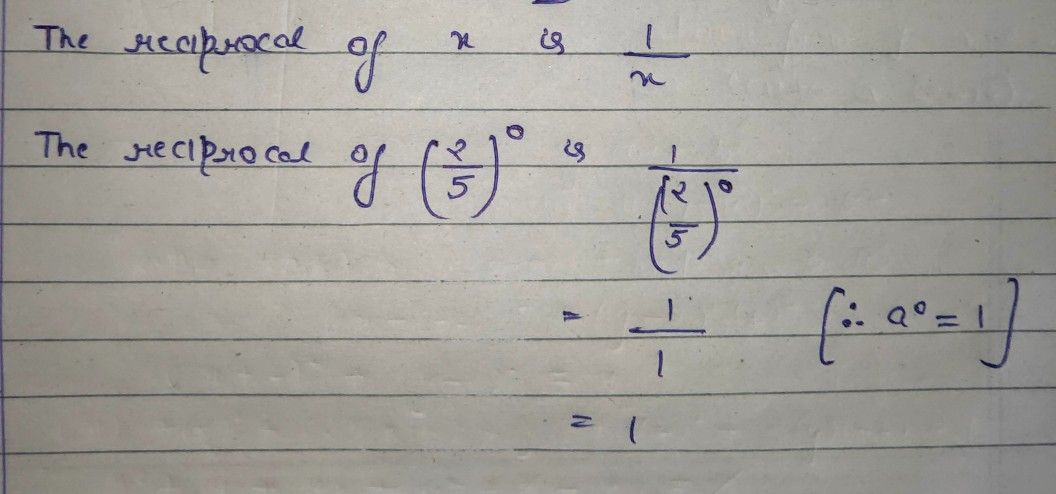Symbol
Problem$8$ $\left(1$ Point) $1\right)$ The\ reciprocal\\ $0+11\right)$ \left(\frac{2} $c\left(2\right)$ {5}\right)^0\ $\right)$ \ $1111s\right)$ $S$ $S1S$ $s3S$ $S4S$ $s2S$
7th-9th grade
Other
SolutionQanda teacher - TanyaThanks for asking a question. You can ask any doubt without any hesitation in a direct way also for high-quality answers quickly and please rate 5 stars if you are satisfied with my answers And, if you have no doubt related to the previous question then please evaluate the answer and give 3000 coins as a gift ( these are not reduced to your wallet)